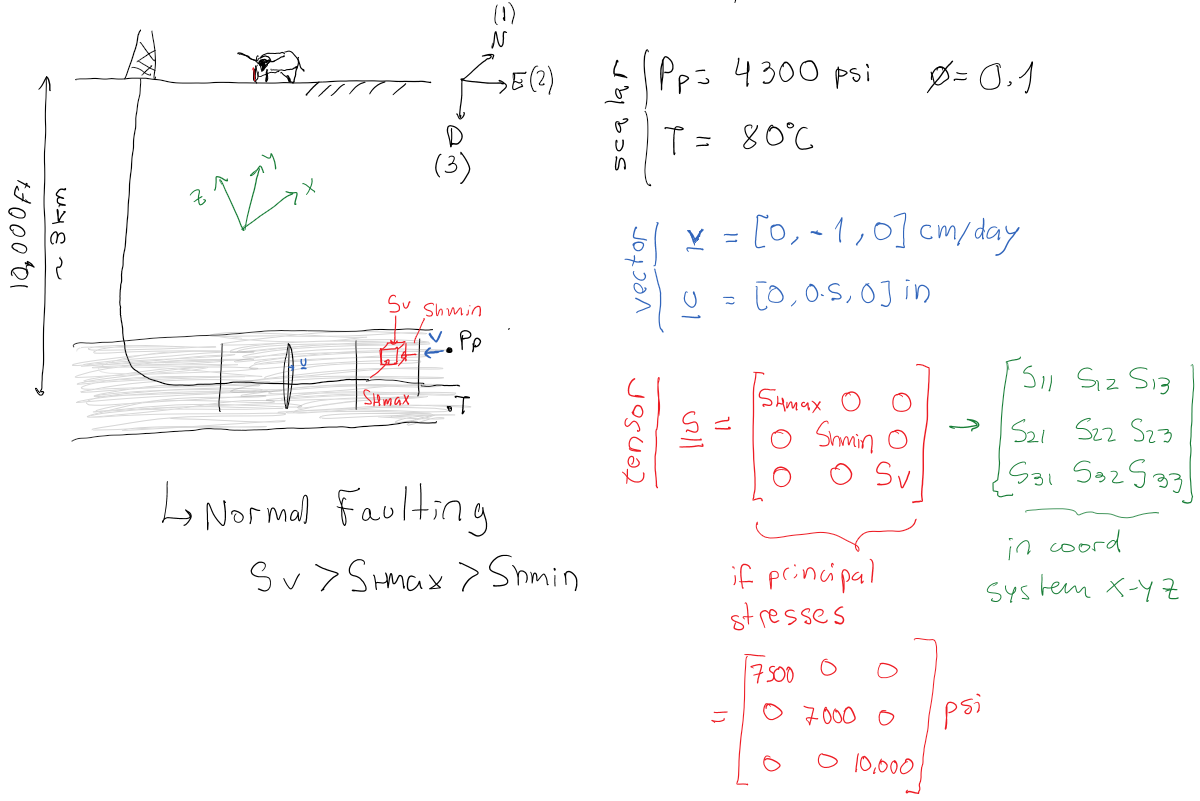Subsections

# 3.1 Stress tensor

Consider a 3D space with a given right-handed orthogonal coordinate system,,in directions 1, 2 and 3 (Figure 3.2). In a right-handed coordinate system, the first element of the baseis your index finger, the second element of the baseis your middle finger, and the third element of the baseis your thumb (all in your right hand).

The number that represents the value of a scalar (such as temperatureor pore pressure) at a given pointis independent of the coordinate system orientation and origin (Figure 3.1). However, the numbers that represent the value of a vector (such as velocityor force) or a tensor depend on the coordinate system. A tensor, like stress, also depends on the coordinate system used to express its numerical values. Read the valuesas the stress on face perpendicular to base vectorin the direction of base vector.is positive if after a displacement,points in opposite direction to(Figure 3.2).All stressescan be written as a matrix (Figure 3.2). The diagonal terms correspond to normal stresses. The off-diagonal terms correspond to shear stresses. Off-diagonal stresses are symmetric() because of angular momentum equilibrium (the element does not spin around any axis). Hence, the stress tensor is symmetric with respect to the diagonal (top-left to bottom-right).Since the stress tensor is symmetric and is composed by all real numbers, there exist 3 real-valued eigenvalues that we call principal stresses and denote. Each principal stress (eigenvalue) is associated with a principal direction (eigenvector). Principal directions are always perpendicular to each other in a cartesian coordinate system. When we write the stress tensor in the coordinate system aligned with directions of the principal stresses, the stress tensor results in diagonal elements populated by the principal stresses and zeros in the off-diagonal places. Usually, the principal stresses are ordered from top to bottom starting withat the top (Figure 3.3).EXAMPLE 3.1: For the following stress tensor obtained for the Neuquen Basin: a) calculate the eigenvalues (principal stresses), b) calculate eigenvectors (principal directions), c) answer what the stress regime is, and d) calculate the angle between the North and the direction ofin clockwise direction.psi

Note: The stress tensor is written in the North-East-Down coordinate system.

SOLUTION
First, find an appropiate math solver that can calculate eigenvalues and eigenvectors (Python, Matlab, Wolfram Alpha, etc.) We will use Wolfram Alpha online in this solution. Go to https://www.wolframalpha.com/ and enter:

eigenvaluesThe answer to this querry is (click in “approximate forms”):anda,b) The principal stresses areFiguring out what are horizontal and vertical stresses depends on the eigenvectors. First, let's start with the easiest one. Eigenvectorpoints straight in the downward direction, i.e., no horizontal component either in N or E directions (first two coordinates are zeros). Hence,is in the vertical direction and is. The other two (and) are the horizontal stresses, whereand therefore.

c)is the intermediate stress in this case, hence, this location is under strike-slip stress regime according to the Andersonian classification.

d) Eigenvectorgives the direction of. Let's read the vectoraccording to the NED coordinate system: it goes North +0.0753277 units, it goes East +1.0 units, it goes Down 0.0 units. Drawing this in a 3D coordinate system results in a vector in the NE horizontal plane pointing mostly towards the East. The angle between the East axis and the vector israd, i.e.,from the East axis towards the North axis. Hence, the angle between the North and the vectoris.## 3.1.1 Cauchy's equations of stress equilibrium

Equilibrium of stresses requires summation of forces in all directions to be zero when the object is not moving (no acceleration, thus). Consider the schematic in Figure 3.4. Summation of forces in direction 1, where the termis the body force component, proportional to the solid mass densityand volume, and the acceleration component, requireswhich eventually reduces to the following equation when canceling terms and dividing by the element volume(3.1)A generalization of equilibrium in all directions with all stresses (Figure 3.2) yields the Cauchy's equilibrium equations:(3.2)

## 3.1.2 Application of Cauchy's equations for total vertical stress calculation

Consider a half-space where the surface coincides with the origin of the coordinate system and gravitypoints in direction 3, hencein Eq. 3.2. We assume infinite extension in directions 1 and 2, therefore there are no variations in directions 1 and 2, such that. Notice there are 6 unknowns and 3 equations in Eq. 3.2 (remember). The only equation we can solve is the third one. Integration of the third equation yields the (vertical) stress,(3.3)

equivalent to Eq. 2.11.You may wonder “what aboutand? The horizontal stresses cannot be determined with the current equations. The solution to this problem will be developed in section 3.3.4.

## 3.1.3 Continuum mechanics solution of an arbitrary problem

Figure 3.6 shows an example of an arbitrary shaped continuous solid subjected to external stresses, external forces, body forces, and displacement constraints (bottom fixture). As highlighted before, notice that there are 6 unknowns (9 unknowns if displacements are included) and 3 equations in Cauchy's equations of equilibrium (Eq. 3.2). The solution of a general problem with arbitrary boundary conditions requires more equations to have a determined problem (as many equations as unknowns). The solution of such problem requires knowledge of the material properties. We need equations that relate displacement to stresses. These equations divide in two types:

• kinematic equations, that relate displacements to strains, and
• constitutive equations, that relate strains to stresses.
The following section describes the simplest form of kinematic and constitutive equations.